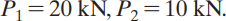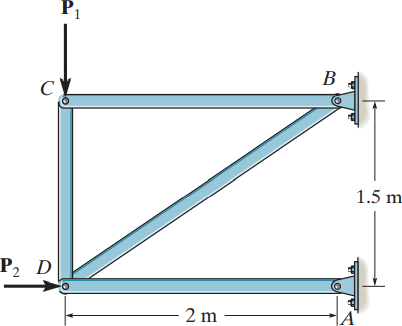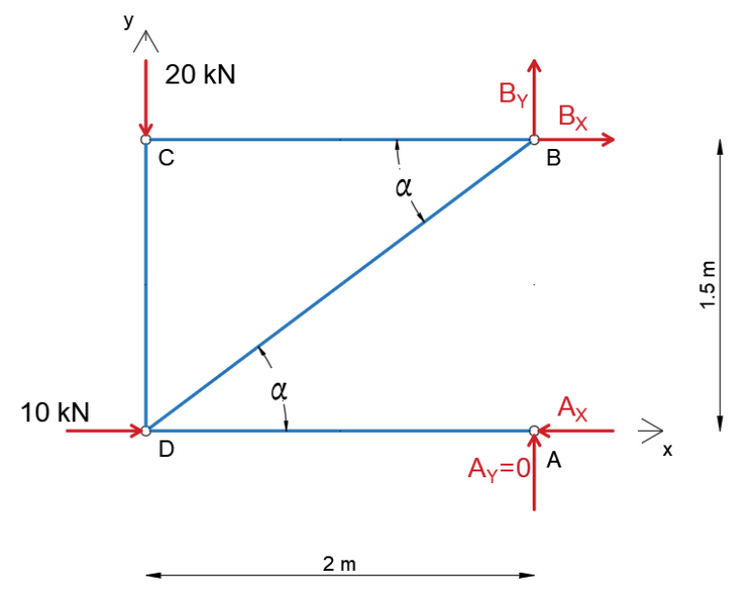×
Get Full Access to Engineering Mechanics: Statics - 14 Edition - Chapter 6 - Problem 6-1
Get Full Access to Engineering Mechanics: Statics - 14 Edition - Chapter 6 - Problem 6-1

×

# Determine the force in each member | Ch 6 - 6-1, 14th EditionISBN: 9780133918922 126

## Solution for problem 6-1 Chapter 6

Engineering Mechanics: Statics | 14th Edition

• Textbook Solutions
• 2901 Step-by-step solutions solved by professors and subject experts
• Get 24/7 help from StudySoup virtual teaching assistantsEngineering Mechanics: Statics | 14th Edition

4 5 1 241 Reviews
27
4
Problem 6-1

Determine the force in each member of the truss and state if the members are in tension or compression. Set P1 = 20 kN, P2 = 10 kN.

Step-by-Step Solution:

Problem 6-1

Determine the force in each member of the truss and state if the members are in tension or compression. SetStep by Step Solution

Step 1 of 11

In order to determine the reaction forces, we’ll need to draw the free-body diagram of the whole system.Step 2 of 11

Step 3 of 11

##### ISBN: 9780133918922

Engineering Mechanics: Statics was written by and is associated to the ISBN: 9780133918922. This textbook survival guide was created for the textbook: Engineering Mechanics: Statics, edition: 14. This full solution covers the following key subjects: compression, determine, Force, member, members. This expansive textbook survival guide covers 11 chapters, and 1136 solutions. Since the solution to 6-1 from 6 chapter was answered, more than 540 students have viewed the full step-by-step answer. The answer to “Determine the force in each member of the truss and state if the members are in tension or compression. Set P1 = 20 kN, P2 = 10 kN.” is broken down into a number of easy to follow steps, and 28 words. The full step-by-step solution to problem: 6-1 from chapter: 6 was answered by , our top Engineering and Tech solution expert on 11/10/17, 05:25PM.

Unlock Textbook Solution